# Divisor

## Table of Contents

 1 Introduction to Divisor 2 What is a Divisor? 3 Difference between Factors & Divisors 4 How to find a Divisor? 5 Important Notes on Divisor 8 Solved Examples on Divisor 9 Thinking out of the Box! 10 Practice Questions on Divisor 11 Maths Olympiad Sample Papers 12 Frequently Asked Questions (FAQs)

We at Cuemath believe that Math is a life skill. Our Math Experts focus on the “Why” behind the “What.” Students can explore from a huge range of interactive worksheets, visuals, simulations, practice tests, and more to understand a concept in depth.

Book a FREE trial class today! and experience Cuemath’s LIVE Online Class with your child.

## Introduction to Divisor

In this article, you will learn about division and its parts.

Division means dividing something in equal parts or groups.

You probably use division in your day to day life and don't even realize it.

For example, let's assume that it is your birthday and you want to distribute a packet of candies among your friends in school.You want to share the candies equally among your friends.

How will you know the number of candies each one will get?To find the answer, you must divide the number of candies by the number of friends.

If there are 32 candies in your packet and you have 8 friends, the mathematical equation for division will be:

$$\bf{32\div8=4}$$

Each of your friends will get 4 candies.Numbers used in division have certain names that describe them.

All 3 numbers have unique names.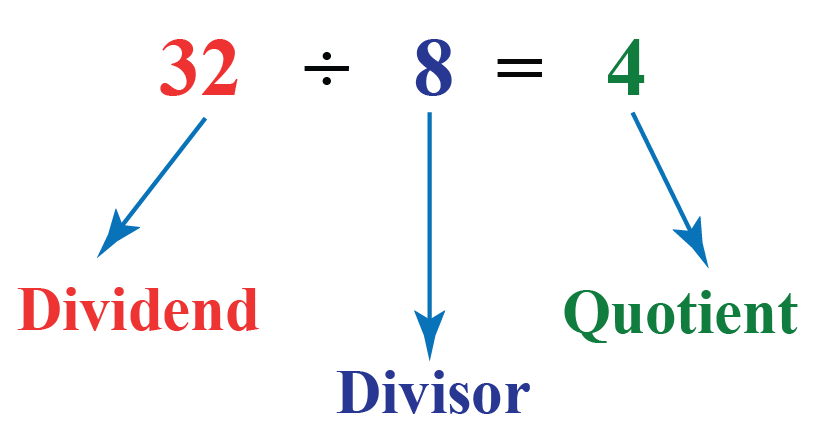In this equation, the number 32 is the dividend, 8 is the divisor and 4 is the quotient.

## What is a Divisor?

### Divisor: Definition in Math

The number we are dividing by is called a divisor.

Divisor takes the dividend and divides it in to smaller parts.

### Divisor and Dividend

The number being divided in a division problem is called a dividend and the number that the dividend divides is called the divisor.

Input the divisor and dividend in the simulation given below by moving the points on the two bars.

You will be able to find out how the divisor divides the dividend.

### Ways to write a division problem

There are 3 different ways to write a division problem.

Don't get confused! All these mean the same thing.

You've already seen one way of writing it.

Let's have a quick look at the other two ways.

The division problem shown below is exactly the same as the one shown above.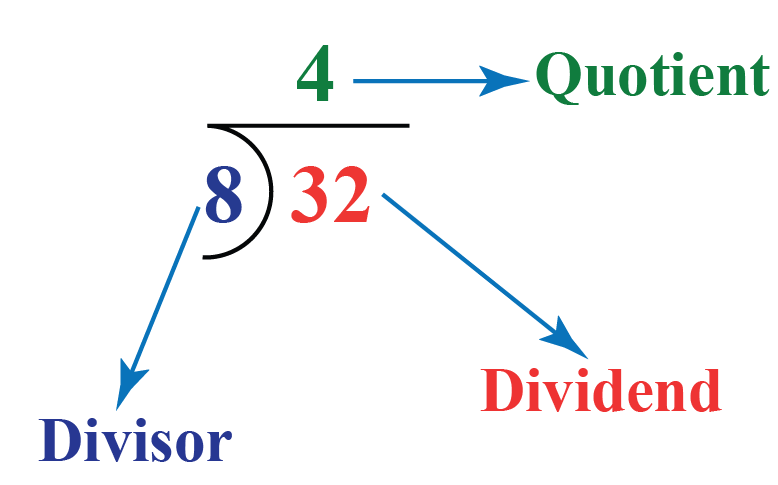The third way to write the division problem is given below.

Again, this is the same as the division problem shown above.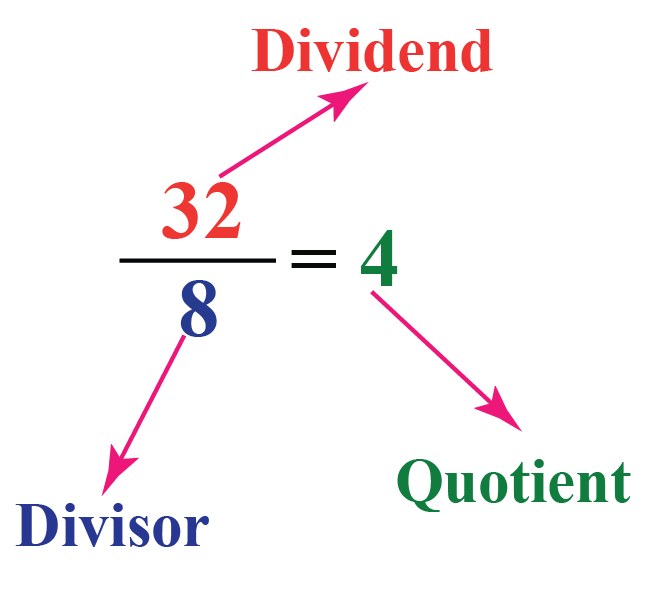## Difference between Factors and Divisors

Divisor is any number that divides the other number whereas a factor is a number that divides the other number and leaves the remainder 0

Thus, all factors are divisors but all divisors are not factors.

The following image shows the factor tree of a number 24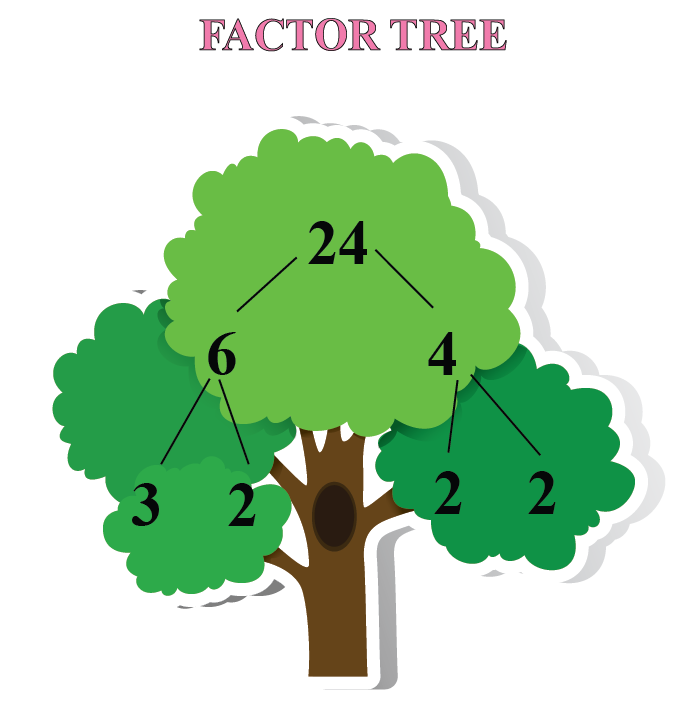Thus, the only factors of 24 are 2 and 3

Let us divide 24 by 5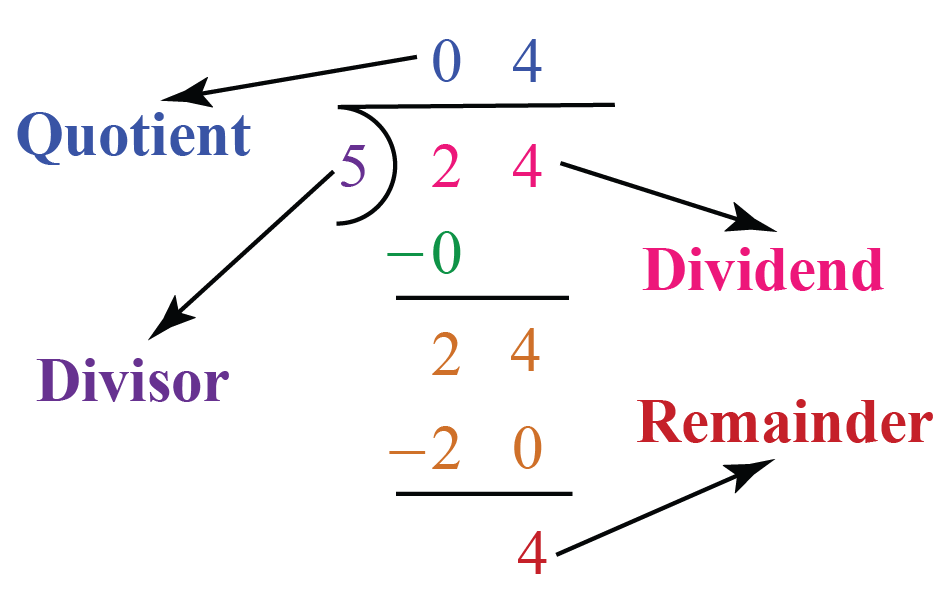Since 5 does not give the remainder 0, it is not a factor of 24

## How to find a Divisor?

Let us recall the different terms of division.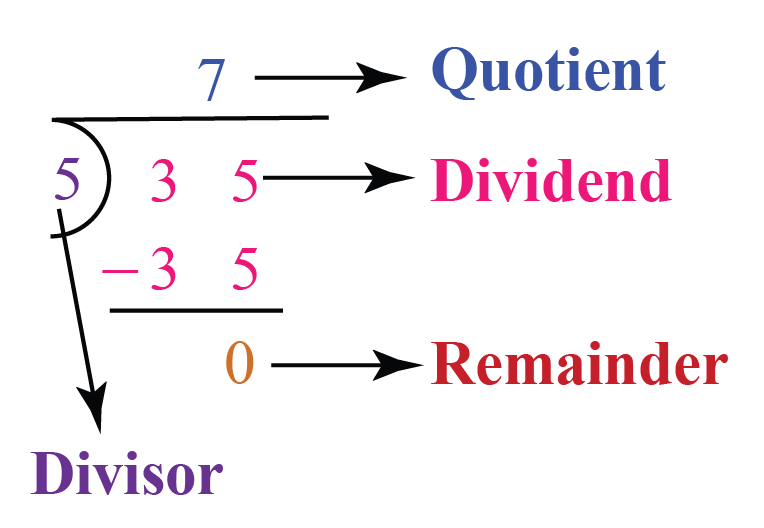The number 35 is the dividend. It is the number to be divided.

The number 5 is the divisor. It is the number that divides the dividend.

The number 7 is the quotient. It is the answer we get after dividing.

The number 0 is the remainder. It is the number which is left over after dividing.

### Divisor: Formula

Remember that if the remainder is 0, then

 $$\text{Divisor}=\text{Dividend}\div\text{Quotient}$$

More Important Topics
Numbers
Algebra
Geometry
Measurement
Money
Data
Trigonometry
Calculus